# Arithmetic progression - math problems

Arithmetic Progression is just a sequence of numbers so that the common difference of any two consecutive numbers is a constant value.

Formula for n-th member is:
${a}_{n}={a}_{1}+\left(n-1\right)\cdot d$

Sum of n AP members:

${s}_{n}=n\cdot \frac{{a}_{1}+{a}_{n}}{2}$

#### Number of problems found: 191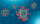A Street has 13 houses in a row. Some resident sin the first house tested positive for Covid-19. The virus spreads in 2 ways: It can spread to the next house or jump directly to the third house. Residents of house 2 can get infected in only one way, house
• GP - sequence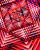The first three terms of a geometric sequence are as follows 10, 30. 90, find the next two terms of this sequence.
• Special sequence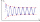What if            2×9=1            3×9=2            4×9=3            5×9=4            6×9=5            7×9=6            8×9=7            9×9=8           10×9=9    then 1×9=??? answer with solutions. .. ..
• 8 wooden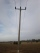8 wooden poles are used for pillers and length of the pillers are from an arithemetric progression if the second pole is 2 metre and the sixth pole in order 5 meters, find the different between the sixth pole and seventh pole.
• HarryHarry Thomson bought a large land in the shape of a rectangle with a circumference of 90 meters. He divided it into three rectangular plots. The shorter side has all three plots of equal length, their longer sides are three consecutive natural numbers. Fi
• Saving in JanuaryOn the 1st of January a students puts $10 in a box. On the 2nd she puts$20 in the box, and so on, putting the same number of 10-dollers notes as the day of the month. How much money will be in the box if she keeps doing this for a the first 10 days of Ja
• Arithmetic progression 2The 3rd term of an Arithmetic progression is ten more than the first term, while the fifth term is 15 more than the second term. Find the sum of the 8th and 15th terms of the Arithmetic progression if the 7th term is seven times the first term.
• The town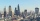The town population is 56000. It is decreasing by 2% every year. What will be the population of the town after 13 years?
• The sidesThe sides of a right triangle form an arithmetic sequence. The hypotenuse is 24 cm long. Determine the remaining sides of the triangle.
• Suppose 3Suppose that a couple invested Php 50 000 in an account when their child was born, to prepare for the child's college education. If the average interest rate is 4.4% compounded annually, a, Give an exponential model for the situation b, Will the money be
• CalculateCalculate the sum of all three-digit natural numbers divisible by five.
• Sequences AP + GPThe three numbers that make up the arithmetic sequence have the sum of 30. If we subtract from the first 5, from the second 4 and keep the third, we get the geometric sequence. Find AP and GP members.
• Squirrels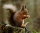The squirrels discovered a bush with hazelnuts. The first squirrel plucked one nut, the second squirrel two nuts, the third squirrel three nuts. Each new squirrel always tore one nut more than the previous squirrel. When they plucked all the nuts from the
• Annual growthThe population has grown from 25,000 to 33,600 in 10 years. Calculate what was the average annual population growth in%?
• Common difference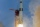The 4th term of an arithmetic progression is 6. If the sum of the 8th and 9th terms is -72, find the common difference.
• Annual increaseThe number of cars produced increased from 45,000 to 47,000 in 3 years. Calculate the average annual increase in cars in%.
• Find the 21Find the sum of the six terms of the finite geometric sequence 96, -48, 24, -12
• Harmonic mean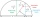If x, y, z form a harmonic progression, then y is the harmonic mean of x and z. Find the harmonic mean of the numbers 6 and 5.
• Harmonic seriesInsert four members between 5/3 and 5/11 to form harmonic series (means).
• Insert 7Insert five harmonic means between 3 and 18

Do you have an exciting math question or word problem that you can't solve? Ask a question or post a math problem, and we can try to solve it.

We will send a solution to your e-mail address. Solved examples are also published here. Please enter the e-mail correctly and check whether you don't have a full mailbox.

Please do not submit problems from current active competitions such as Mathematical Olympiad, correspondence seminars etc...